# Class 8 Maths NCERT Solutions for Chapter – 6 Squares and Square Roots Ex – 6.4

## Squares and Square Roots

Question 1.
Find the square root of each of the following numbers by Division method :
(i)
2304
(ii) 4489
(iii) 3481
(iv) 529
(v) 3249
(vi) 1369
(vii) 5776
(viii) 7921
(ix) 576
(x) 1024
(xi) 3136
(xii) 900

Solution: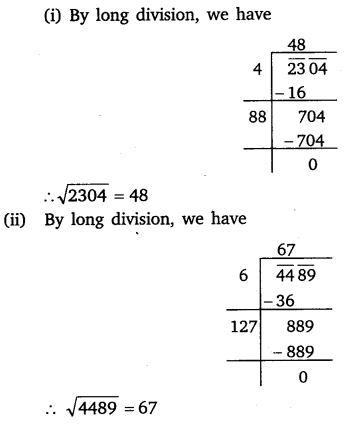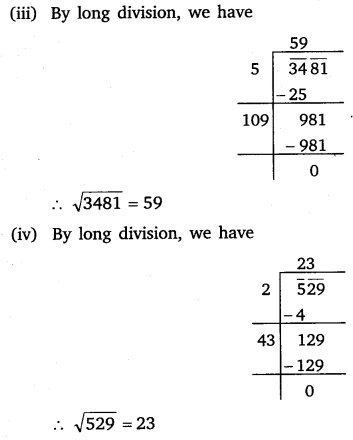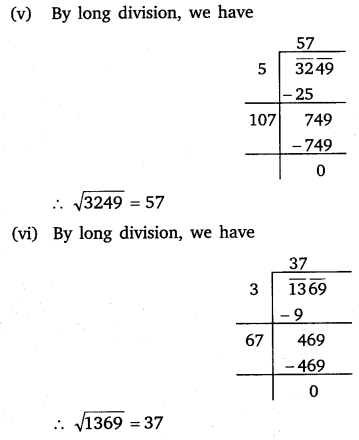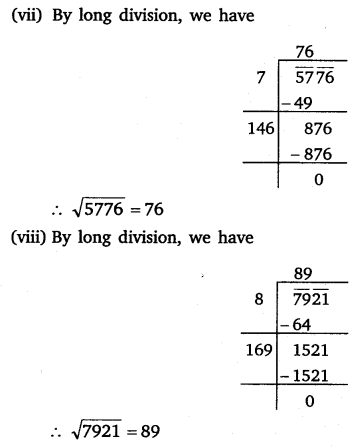Question 2.
Find the number of digits in the square root of each of the following numbers (without any calculation) :
(i)
64
(ii) 144
(iii) 4489
(iv) 27225
(v) 390625

Solution:Question 3.
Find the square root of the following decimal numbers :
(i)
2.56
(ii) 7.29
(iii) 51.84
(iv) 42.25
(v) 31.36

Solution:
(i) Here, the number of decimal places is already even. So, mark off periods and proceed as under :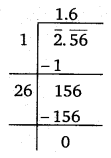∴$\sqrt { 2.56 } =1.6$

(ii) Here, the number of decimal places are already even. So, mark off periods and proceed as under :∴$\sqrt { 7.29 } =2.7$

(iii) Here, the number of decimal places are already even. So, mark off periods and proceed as under :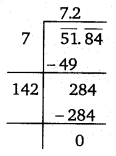∴$\sqrt { 51.84 } =7.2$

(iv) Here, the number of decimal places are already even. So, mark off periods and proceed as under :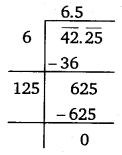∴$\sqrt { 42.25 } =6.5$

(v) Here, the number of decimal places are already even. So, mark off periods and proceed as under :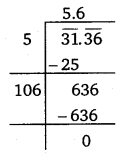∴$\sqrt { 31.36 } =5.6$

Question 4.
Find the least number which must be subtracted from each of the following numbers so as to get a perfect square. Also find the square root of the perfect square so obtained.
(i)
402
(ii) 1989
(iii) 3250
(iv) 825
(v) 4000

Solution:
(i) Let us try to find the square root of 402.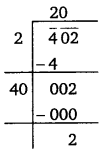This shows the (20)2 is less than 402 by 2. So, in order to get a perfect square, 2 must be subtracted from the given number.
∴ Required perfect square number = 402 – 2 = 400
Also,$\sqrt { 400 } =20$

(ii) Let us try to find the square root of 1989.This shows that (44)2 is less than 1989 by 53. So, in order to get a perfect square, 53 must be subtracted from the given number.
∴ Required perfect square number = 1989 – 53 = 1936
Also,$\sqrt { 1936 } =44$

(iii) Let us try to find the square root of 3250.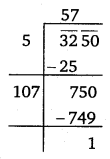This shows that (57)2 is less than 3250 by 1. So, in order to get a perfect square, 1 must be subtracted from the given number.
∴ Required perfect number = 3250 -1 = 3249
Also,$\sqrt { 3249 } =57$

(iv) Let us try to find the square root of 825.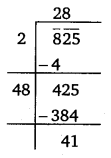This shows that (28)2 is less than 825 by 41. So, in order to get a perfect square, 41 must be subtracted from the given number.
∴ Required perfect square number = 825 – 41 = 784
Also,$\sqrt { 784 } =28$

(v) Let us try to find the square root of 4000.This shows that (63)2 is less than 4000 by 31. So, in order to get a perfect square, 31 must be subtracted from the given number.
∴ Required perfect square number = 4000 – 31 = 3969
Also,$\sqrt { 3969 } =63$

Question 5.
Find the least number which must be added to each of the following numbers so as to get a perfect square. Also find the square root of the perfect square so obtained.
(i)
525
(ii) 1750
(iii) 252
(iv) 1825
(v) 6412

Solution:
(i) We try to find out the square root of 525.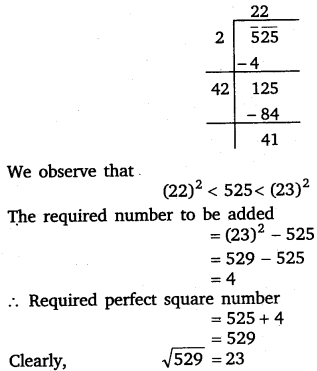(ii) We try to find out the square root of 1750.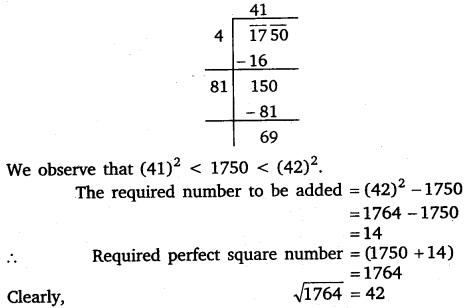(iii) We try to find out the square root of 252.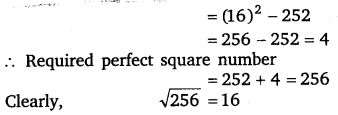(iv) We try to find out the square root of 1825.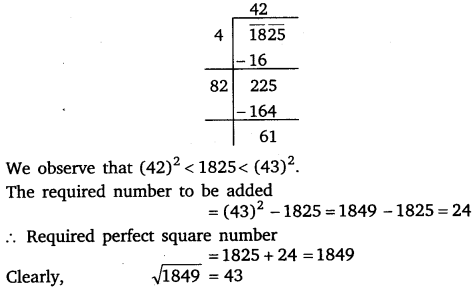(v) We try to find out the square root of 6412.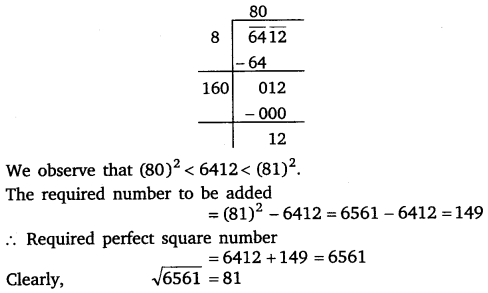Question 6.
Find the length of the side of a square whose area is 441 m2.

Solution: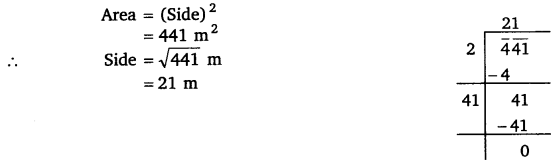Question 7.
In a right triangle ABC, ∠B = 90°.
(a)
If AB = 6 cm, BC = 8 cm, find AC.
(b) If AC = 13 cm, BC = 5 cm, find AB.

Solution: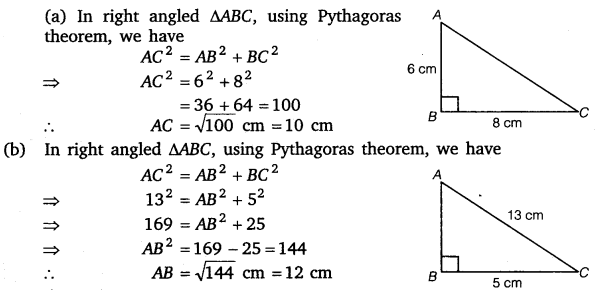Question 8.
A gardener has 1000 plants. He wants to plant these in such a way that the number of rows and the number of columns remain same. Find the minimum number of plants he needs more for this.

Solution:
Let us find the square root of 1000.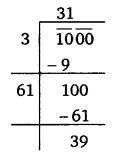This shows that (31)2 is less than 1000 by 39 and (32)2 =1024. Thus, the gardener needs 1024 -1000 = 24 plants more to plant in such a way that the number of rows and the number of columns remain the same.

Question 9.
There are 500 children in a school. For a P.T. drill they have to stand in such a manner that the number of rows is equal to number of columns. How many children would be left out in this arrangement?

Solution:
Let us find the square root of 500.
This shows that (22)2 = 484 is less than 500 by 16.
∴ 16 students have to go out for others to do the P.T. practice as per condition.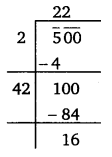### Take Your IIT JEE Coaching to Next Level with Examtube

• Mentoring & Teaching by IITians
• Regular Testing & Analysis
• Preparation for Various Engineering Entrance Exams
• Support for School/Board Exams